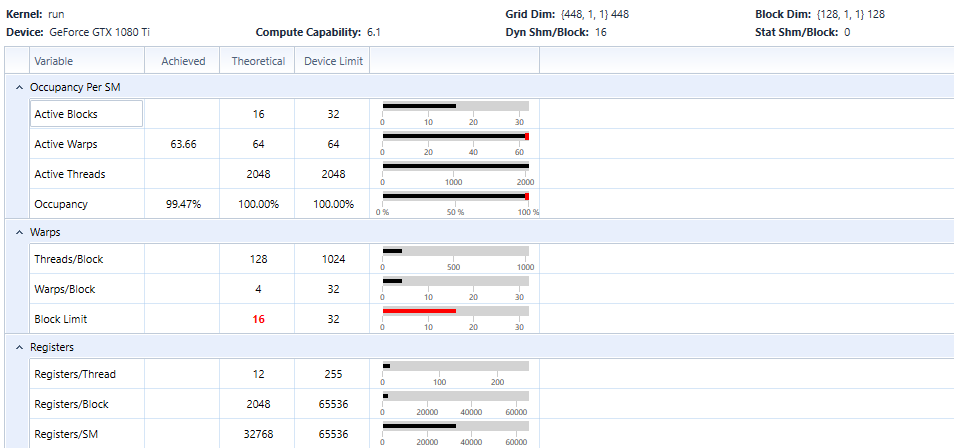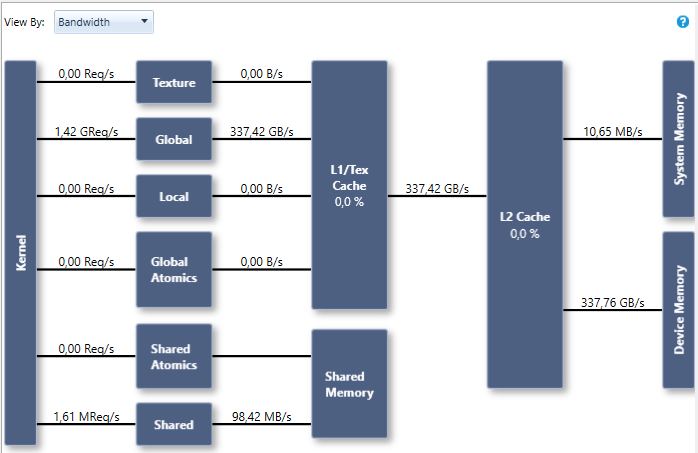### Hybridizer HOWTO — Hello World

Our hello world is the addition of two vector of elements. The C# code is downloadable from our github.

### Hello World : simple work distribution

We start with a simple way to express parallelism: the `Parallel.For` construct, which is natively proposed by .Net. We place the `EntryPoint` attribute on the method tro trigger hybridization:

```[EntryPoint]
public static void VectorAdd(double[] a, double[] b, int N)
{
Parallel.For(0, N, (i) => { a[i] += b[i] });
}
```

As usual, we need to invoke this method with some boilerplate code.

### Hello World : explicit work distribution

We can also use explicit work distribution, which is done using a CUDA-like syntax: `threadIdx/blockDim`, `blockIdx/gridDim`. This is customizable and names can be changed, but the concept is similar:

```
[EntryPoint]
public static void VectorAdd(double[] a, double[] b, int N)
for (int k = threadIdx.x + blockDim.x * blockIdx.x ;
k < count ; k += blockDim.x * gridDim.x)
{
a[k] += b[k];
}
}
```

Explicit work distribution can be used (for example) to distribute work among a 2D-grid.

### Grid configuration

To achieve hich bandwidth, we need to properly configure the grid. Using enough blocks and threads increases occupancy and can mask latency by running concurrent blocks. We do that as we would in CUDA:

```cudaDeviceProp prop;
cuda.GetDeviceProperties(out prop, 0);
HybRunner runner = HybRunner.Cuda("HelloWorld_CUDA.dll").SetDistrib(prop.multiProcessorCount * 16, 128);
```

### Performance measurements

We can now compile this in Release|x64 and profile the execution with nsight. We reach very high occupancy:We reach 337.8GB/s on a GTX 1080Ti (Pascal), which is 96% of bandwidth test on this GPU:Tags: ,